# Hashing and its Use Cases in Java

Challenge Inside! : Find out where you stand! Try quiz, solve problems & win rewards!
Learn via video courseJava Course - Mastering the Fundamentals
By Tarun LuthraFree
5Enrolled: 1000Java Course - Mastering the Fundamentals
Tarun LuthraFree
5
Enrolled: 1000

## Overview

Hashing in Java is a technique for mapping data to a secret key, that can be used as a unique identifier for data. It employs a function that generates those keys from the data; this function is known as the Hash-function, and the output of this function (keys) is known as Hash-values. To provide quick access to the data, we store it as key(Hash values) value(real data) pairs in an array called Hash-table, which uses keys as indexes and values as the data to be stored at each index location.

## Introduction

We've all used hashing in our daily lives; remember how in university, we were all identified by a unique number called our Roll number? In this case, the Roll number serves as the key, and the student details (name, address, course, age, etc.) serve as the values, and we can easily store this data in an array where the Roll number serves as the index to store the associated details.

However, Hashing can be used when the keys are large or non-integer and cannot be used directly as an index. Hashing in java is primarily the process of converting a given key (roll no) into another value (array indexable integer in this case).

Firstly, hashing in java or in any other language uses a Hash function to convert given keys (Roll no) into some other useful value, called hash-value, based upon the requirement a hash-value can be an integer to be used as an array index, a secret code for some password etc... Then, the associated data (student details associated with particular roll no) is stored in the hash table in the form of <hash-value, associated data> pair.

## What is Hashing in Java?

Hashing in Java is the technique that enables us to store the data in the form of key-value pairs, by modifying the original key using the hash function so that we can use these modified keys as the index of an array and store the associated data at that index location in the Hash table for each key.

### Hash Function

While implementing hashing in java it uses a function called hash function, it is the most important part of hashing; it transforms supplied keys into another fixed-size value (hash-value). The value returned by a hash function is called hash value, hash code, or simply hashes.

•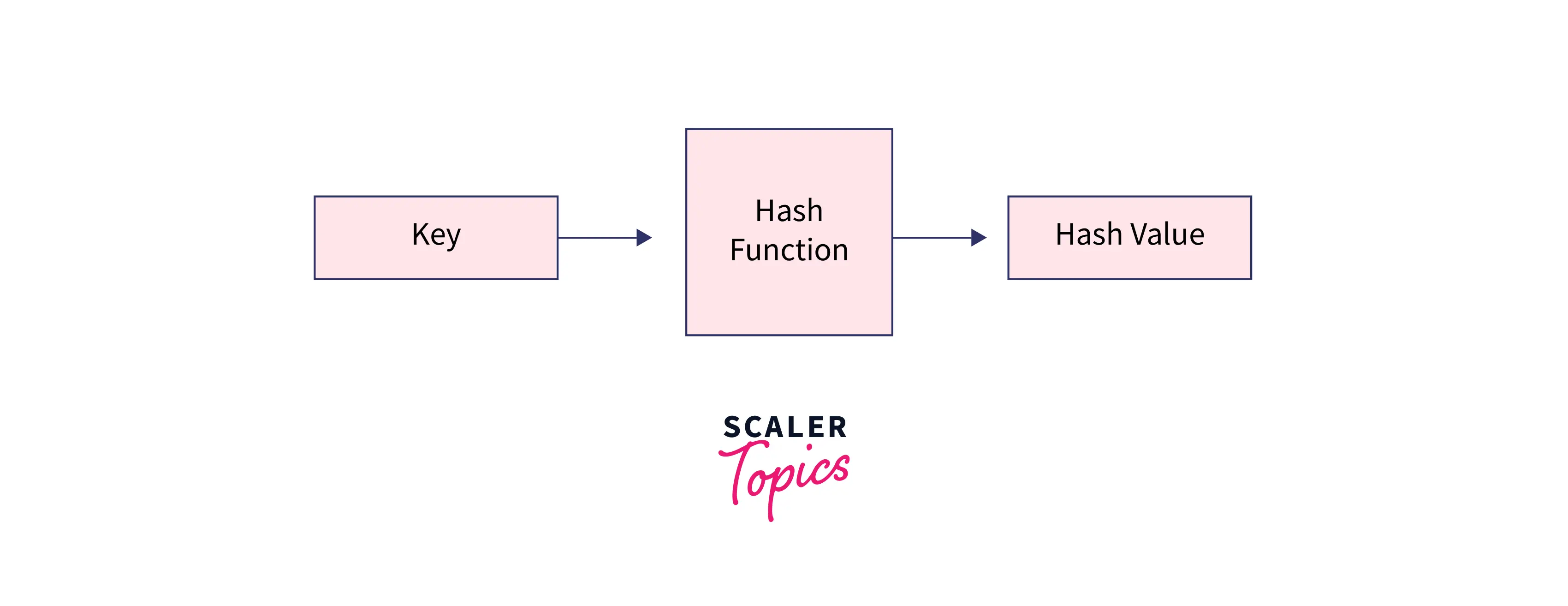### Hash table

A hash table is an array that holds pointers to data that corresponds to a hashed key. Hash table uses hash values as the location index to store the associated data in the array.

•Basically, the given keys are converted into hash values using a hash function and these hash values are used as the index of the hash table to store the associated data.

Hashing in java can be termed as the entire process of storing data in a hash table in the form of key-value pairs, with the key computed using a hash function.

## Characteristic of a Hashing Algorithm

The hashing algorithm is used for generating fixed-length numeric hash-value from the input keys. We anticipate our hash function to have the following features because we created hashing for quick and efficient core operations.

• It should be very fast on its computation and should convert the given key into a hash value quickly.

• The algorithm should avoid generating a hash value from a message's (input) generated hash value (one way).

•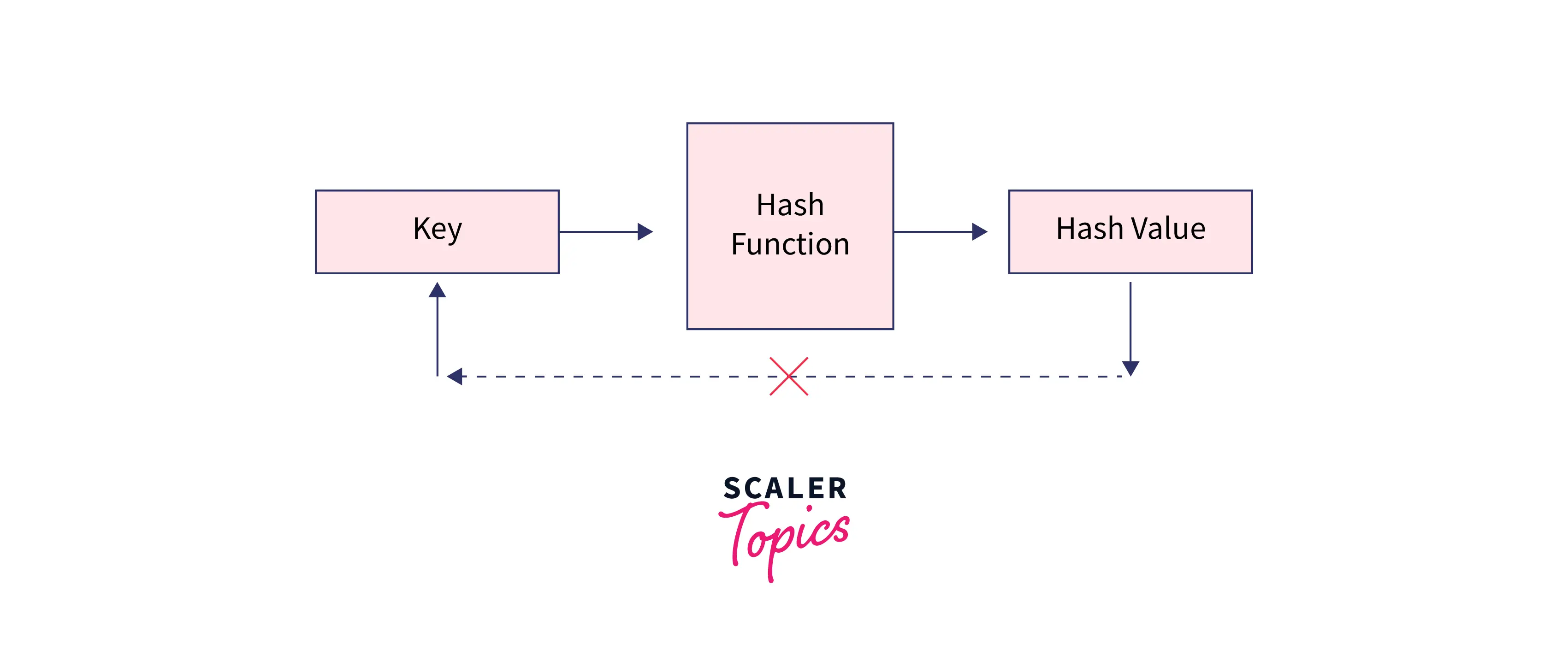• Hashing algorithm must avoid the collision. Actually, a collision occurs when two differed inputs to the hash function are converted to the same hash value.

•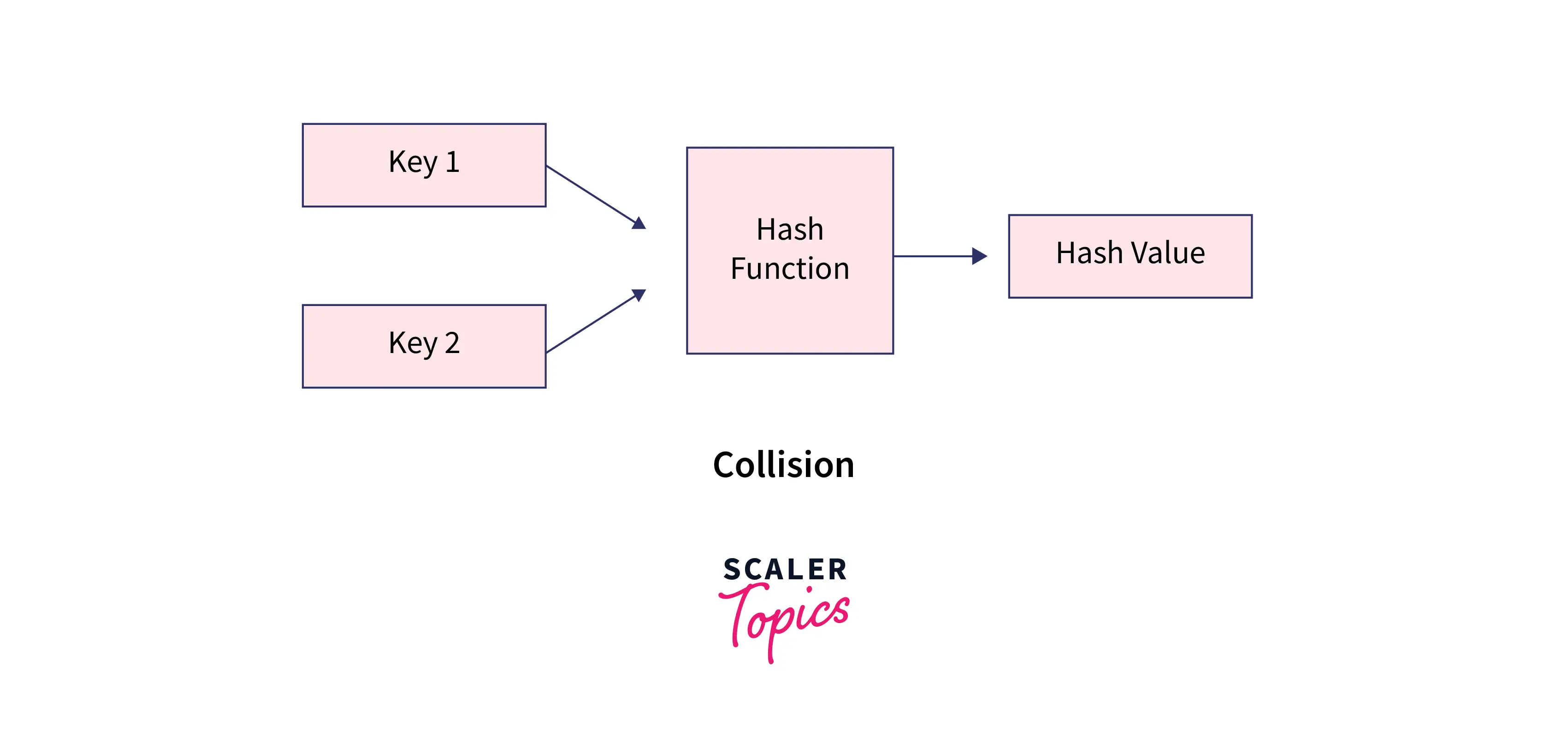• When the message's hash value changes even slightly, the message's hash value must change as well. The avalanche effect is what it's called.

## How does Hashing Work?

Hashing in java is a two-step process:

• firstly, A hash function is used to turn an input key into hash values.

• This hash-value is used as an index in the hash table and corresponding data is stored at that location in the table.

•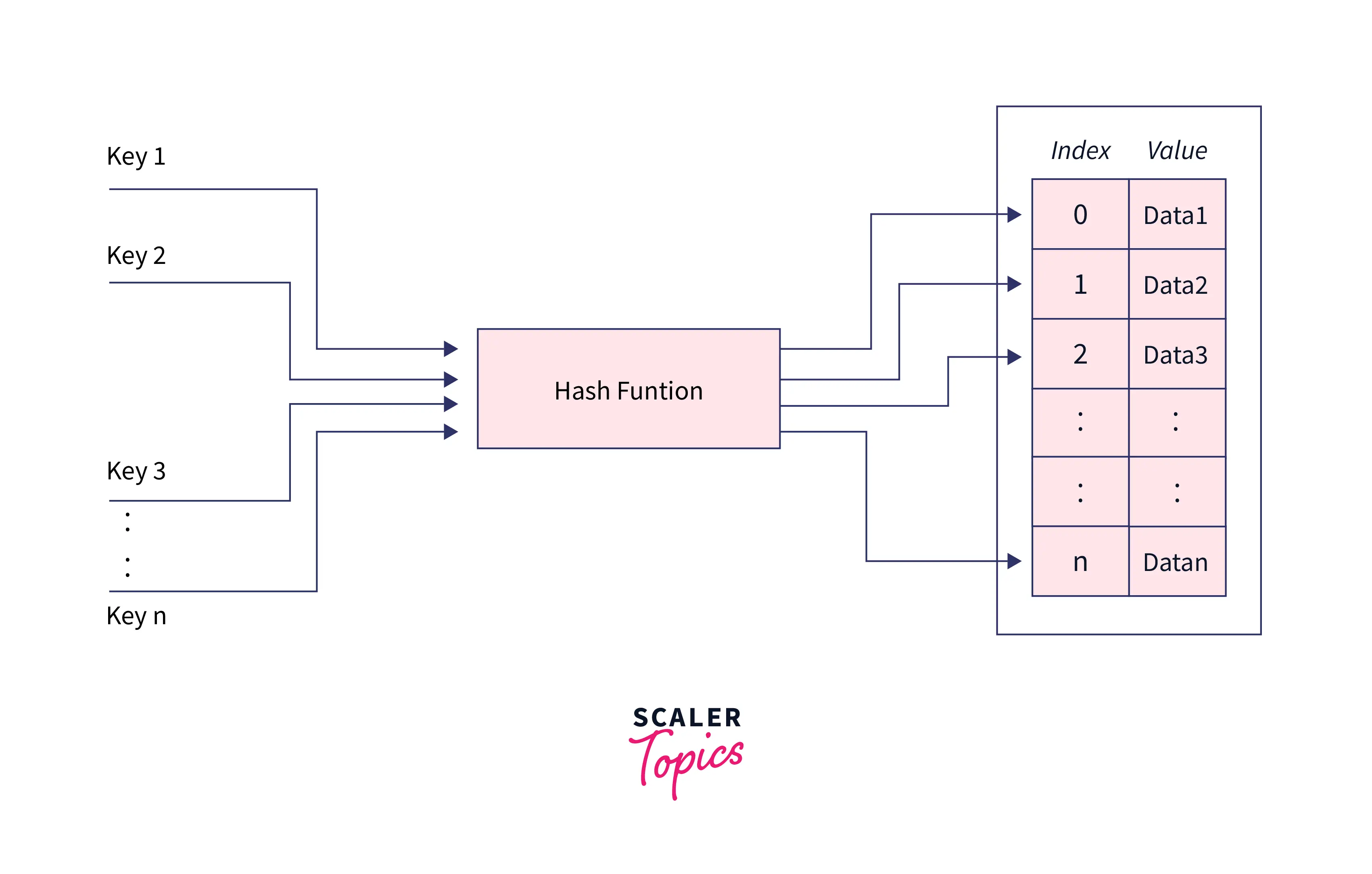• The element is stored in a hash table and can be retrieved quickly using the hashed key. In order to retrieve data from the hash table, we first calculate the hash value for a given key and as we know this key was used as an index while storing data in the hash table so we extract the data from that index.

To better understand how hashing works, consider the following scenario: we are given the token number and the name of the people who will be vaccinated today. The person is uniquely identified by token no.

The table below shows contains the name and token numbers as stated above.

Token No.Name
16Virat
1Alex
40Ishika
5Sonu
3Mrinalini
38John

Our goal is to create a quick and space-efficient hash table for data storage and retrieval.

To solve this problem, one nieve solution we can think of is to use an array of size 41 so that we can be able to use each key(token no) as the index to the array and store the data at those index locations.

This works but it is inefficient and we will be wasting the majority of the space we have used because we will be having data stored at only six (1,5,3,16,38 and 40) locations out of 41. we should think of a method to narrow down the search space for us.

In such cases, we can use hashing, We can use think of a function that is able to convert the given keys (token no) into less spread hashed keys and follows all the characteristics of the hash function discussed in the section above.

Choosing hash function

If we look closely at the keys, we can see that they can easily be converted to numbers from 0 to 10 if we use

Hash(key) = key%10.

Using this hash function we can observe that

• Hash(16) = 16%10 = 6, indicating that the value corresponding to key 16 i.e. (virat)will be stored in the array at index 6.

• Similarly other keys can be hashed in the same way to find a suitable location in the array.

• Our hash table should be of size 10 because the hash function can be able to give hash values from 0 to 9.

Token No.NameHash(key) = key%10
16Virat16%10 = 6
1Alex1%10 = 1
40Ishika40%10 = 0
5Sonu5%10 = 5
3Mrinalini3%10 = 3
38John38%10 = 8

So our hash-table will look like:

Index0123456789
ValueIshikaAlexMrinaliniSonuViratJohn

Now if we want to be able to get the name of the person with token no 38, then we can generate an index using the hash function we used above.

index = hash(38) = 38%10 = 8.

and we can get the value from the array now, table will return John as the name of that person.

Now suppose we have one more person's data with token no 23, in that case, the hash function we used above will generate the same hash values for both 23 and 3. This is what we call collision when two different keys are converted to the same hash value by the hash function. We have no. of techniques to resolve collision.

Chaining: Although the hash function should minimize the collision, if it still happens then we can use an array of LinkedList as a hash table to store data, the fundamental idea is for each hash table slot to point to a linked list of records with the same hash value. This technique is called chaining type of hashing in java.

•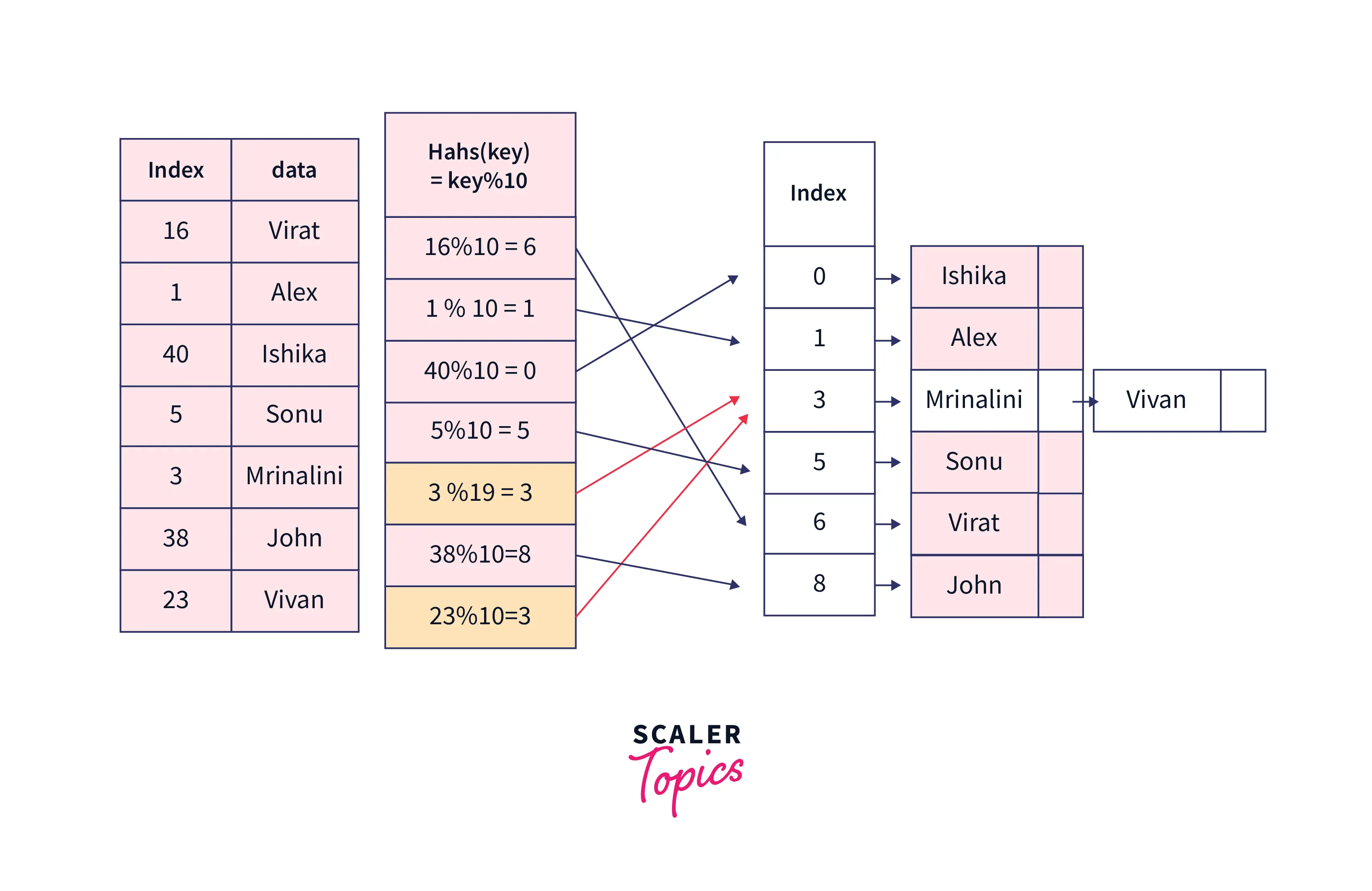Double Hashing in java: As the name suggest, it uses two hash functions to resolve collision, we employees 2nd hash function wherever collision occurs.

•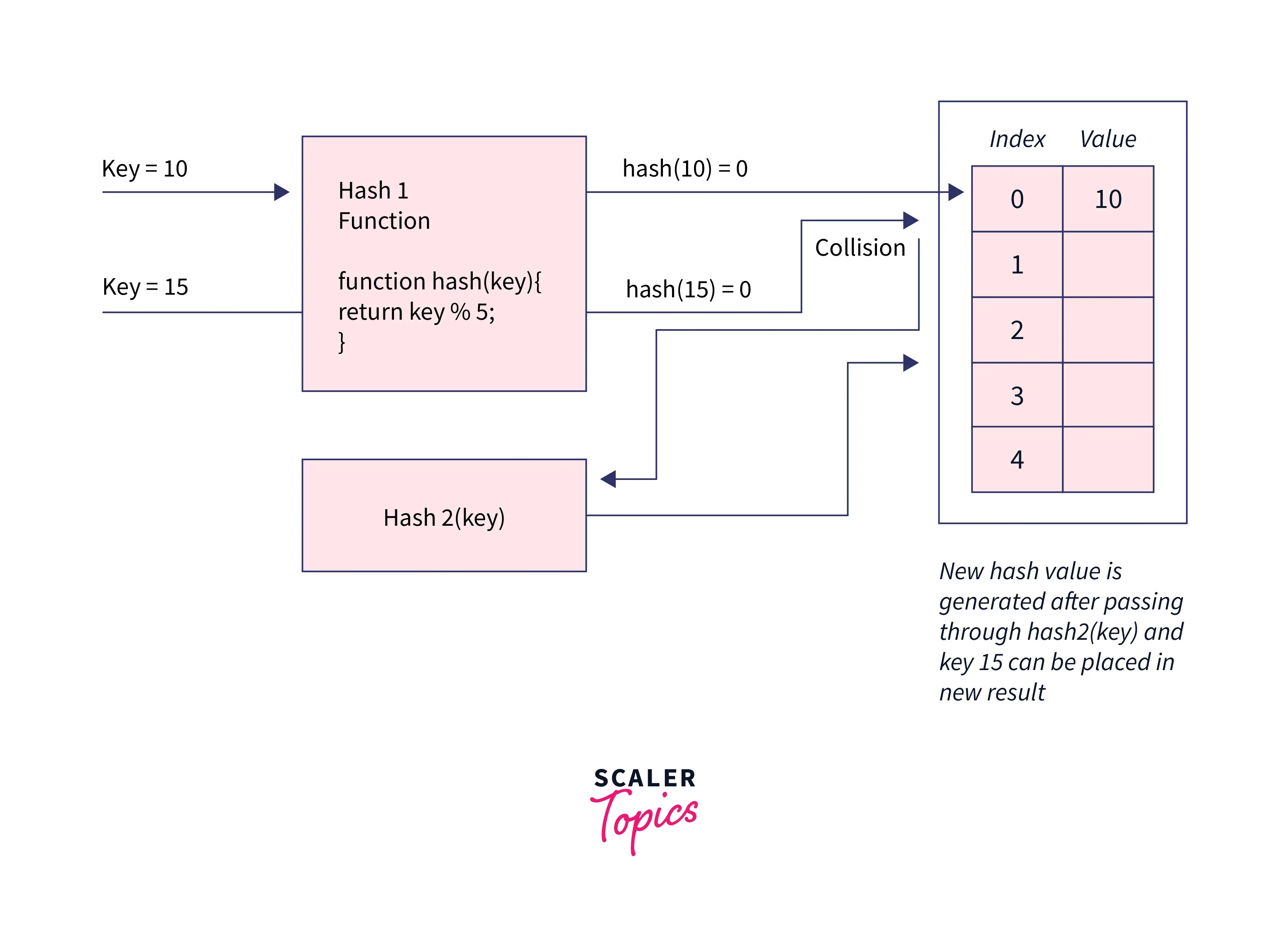## Types of Hashing Algorithms

Hashing in java implements some algorithm to hash the values, a few algorithms are -

### MD5 Algorithm

The Message-Digest Algorithm (MD5) is a widely used cryptographic hash function that generates a hash value of 128 bits. The main idea is to map data sets with variable lengths to data sets with constant lengths. It is a data verification algorithm used in transmission protocols. The MD5 hash is used in many web applications to prevent security breaches, hacking, and other issues.

To accomplish the hash-value (message digest), the input message is divided into 512-bit blocks. Padding is attached to the end of the piece in such a way that its size can be divided by 512. The blocks are now processed using the MD5 algorithm, which operates at 128 bits, yielding a 128-bit hash value. The generated hash is usually a 32-digit hexadecimal number after applying the MD5 algorithm. The string to be encoded is referred to as the message, and the hash value generated after hashing is referred to as the digest or message digest.

### SHA Algorithms

Secure hash algorithm (SHA) is also one type of Cryptographic function. The algorithm is similar to MD5. The SHA algorithm, on the other hand, produces much stronger hashes than the MD5 algorithm. The SHA algorithm generates hashes that aren't always unique, which means there's a chance of collision. SHA, on the other hand, has a much lower collision rate than MD5. The output of SHA is called hashcode, there are four types of SHA algorithms based upon the size of the hash generated.

• SHA-1 - It is the most basic SHA. It generates a hashcode of 160 bits.

• SHA-256 - It has a higher level of security than SHA-1. It generates a hash with a length of 256.

• SHA-384 - SHA-384 is a one-level higher than SHA-256, with a 384-bit hash.

• SHA-512 - It is the most powerful of all the SHAs mentioned. It generates a 512-bit hash.

### PBKDF2WithHmacSHA1 Algorithm

The main goal of the PBKDF2WithHmacSHA1 algorithm is to slow down the brute force attack and reduce the damage. The goal is to make the hash method as slow as possible so that the attacks are delayed. At the same time, it must be fast enough to avoid causing the user any significant delays in generating the hash. As an argument, the algorithm takes a security factor (also known as work factor) or iteration count. The hash function's slowness is determined by the work count value. The higher the value of this iteration count or work factor, the more efficient the hardware.

## Use Cases of Hashing in Java

Hashing in java is very easy because of in-built classes, here we will see some examples. But before that let's quickly go over some terminologies.

• Synchronization in java it is the capability to control the access of multiple operation to any shared resource. If the given source is non-synchronized that it can be used in multithread environment then more than one thread can access and process the given source simultaneously.

•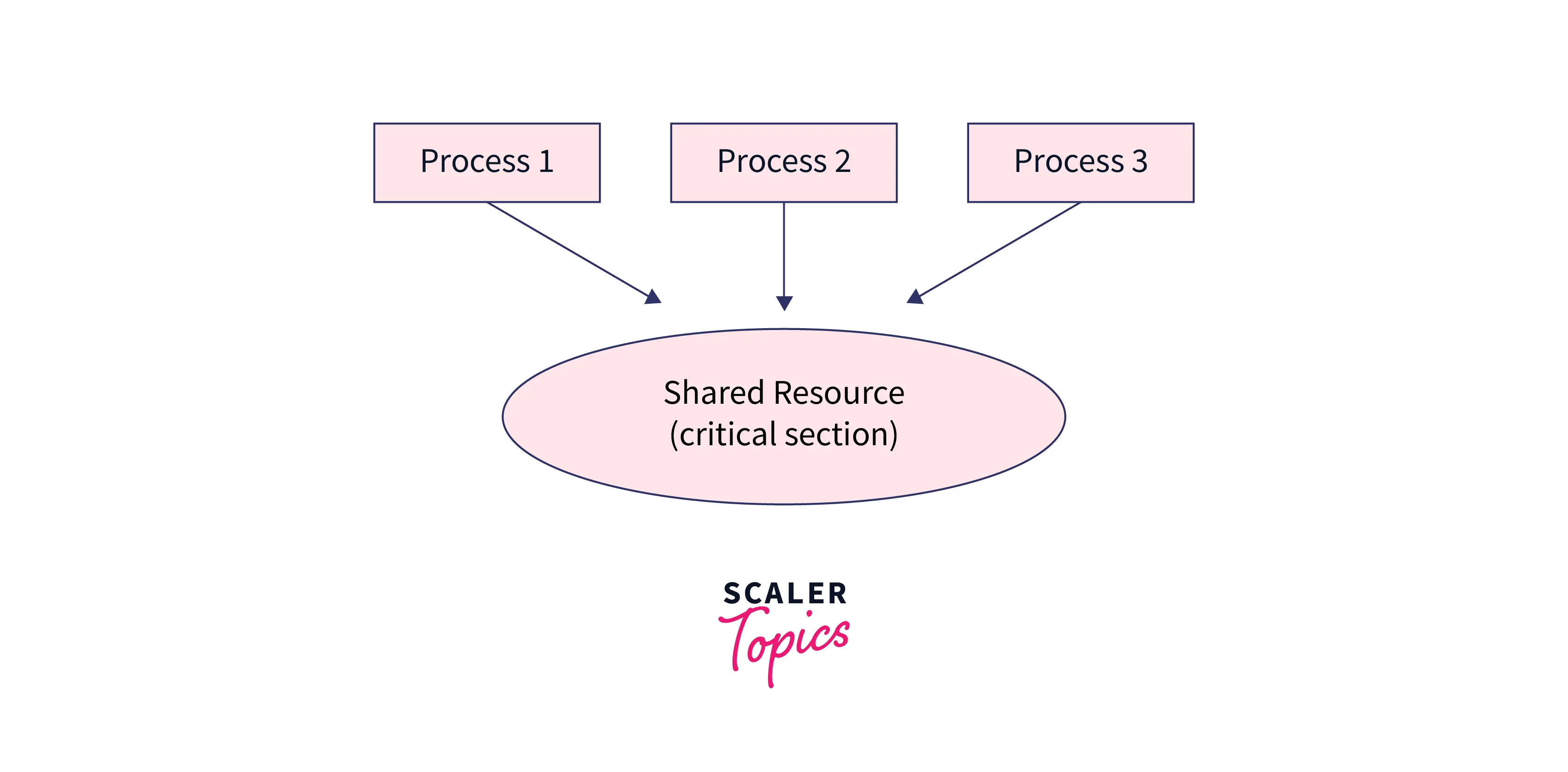Non-synchronized

• A synchronized source can not be accessed by multiple processes simultaneously, it needs to finish one processes before moving to another one.
• Load factor : The load factor is a measurement of how full a hash table can get before its capacity is increased automatically. Hashing in jave uses it as an indicator to increase the size of hash table.

### HashTable

Hashtable in java is an in-built class, it creates a hashtable by mapping keys to values. It implements the Map interface and inherits from the Dictionary class.

• A Hashtable is a list's array. Every list is referred to as a bucket. The hashcode() method is responsible for hashing input key to get an integer that can be used as an index in the array to determine bucket's position. A Hashtable is a collection of values based on a key.
• The hashtable class in java has been synchronised. It prevents multiple threads from accessing the Hashtable at the same time.
• Java Hashtable class contains unique non Null (key or values) elements.
• It does not allow you to use duplicate keys, if you try to do that then latest instant of duplicate key value pair is stored, in other words previous values are overwritten.
• The default capacity of Hashtable class in Java is 11 whereas loadFactor is 0.75.

Declaration:

Type Parameters:

K – data the type of keys maintained by this map

V – data the type of mapped values

Constructors to create hash table:

• Hashtable(): This creates an empty hashtable with the default load factor of 0.75 and an initial capacity is 11.

• ```Hashtable<K, V> HT = new Hashtable<K, V>(int initialCapacity = 11, float fillRatio = 0.75);
```
• we can costumize the values of capacity and load factor by passing them to the constructor Hashtable(), if we dont it will consider defalut values.

Using the code below we are aiming to create a hash table for the same vaccination slots data (token no and name) example we used earlier. We will create hashtable using Hashtable constructor and add data using put mathod and finally will extract data using getkey() and getvalue() methods.

Output

### HashMap

HashMap, also known as HashMap<Key, Value> or HashMapK, V>, is an easy way to implement hashing in java, it is a Map-based collection class that is used to store key-value pairs. It uses an array and LinkedList data structure internally for storing Key and Value.

• It uses hashCode() method of object class, which implements hashing and returns the index of the object called hash-value or hash. This hash is used as bucket no that is the address of element inside the map.

• The order of the map is not guaranteed by this class.

• It's similar to the Hashtable class, but it's unsynchronized and allows null values (null values and null key).

• HashMap does not allow duplicate keys to be stored. If you try to store a duplicate key with a different value, the value will be replaced. Which indicates that we can at max have one NULL key in the map.

• It has an initial capacity of 16 and load factor of 0.75.

Declaration:

Type Parameters:

K – data the type of keys

V – data the type of mapped values

Constructors to create hashmap:

• HashMap(): This creates an empty hashtable with the default load factor of 0.75 and an initial capacity is 16.

• ```HashMap<K, V> HM = new HashMap<K, V>(int initialCapacity = 11, float fillRatio = 0.75);
```
• we can costumize the values of capacity and load factor by passing them to the constructor HashMap(), if we dont it will consider defalut values.

Code below how we can hashmap and put data in it, we are using the same example again. We will delete elements using remove(key) and remove(key,value) methods and at the end we will print our hashmap.

Output

The Map interface is implemented as a Hashtable and Linked list in Java's LinkedHashMap class, with predictable iteration order. It inherits the HashMap class and implements the Map interface. This implementation, unlike HashMap, keeps a doubly-linked list running through all of its entries. The iteration ordering, which is typically the order in which keys are added to the map, is specified by this linked list (insertion-order).

• It stores unique elements.
• It may have one NULL key and multiple different keys with NULL values.
• It stores data in the form of key value pair and all the input keys are converted into a hash value using hashing technique, similar to the HashMap.

Declaration

• LinkedHashMap(): similar to hashmap, it creates an empty LinkedHashMap with the default load factor of 0.75 and an initial capacity is 16.

• ```LinkedHashMap<K, V> HM = new LinkedHashMap<K, V>(int initialCapacity = 11, float fillRatio = 0.75);
```
• we can costumize the values of capacity and load factor by passing them to the constructor LinkedHashMap(), if we dont it will consider defalut values.

The code below is showing us the implementation of syntax for the same example, we create the data structure using constructor and add data to it using put() and finally iterate through it and extract keys and values.

Output

Other operation like remove, get (for getting values by key) works same as previous two.

### Concurrent HashMap

The Java collections framework's ConcurrentHashMap class provides a thread-safe map. That is, multiple threads can access the map at the same time without affecting the map's consistency. The ConcurrentMap interface is implemented. It stores key value pairs.

• Concurrency-Level: It is the number of threads concurrently updating the map. The implementation performs internal sizing to try to accommodate this many threads.
• The Object in ConcurrentHashMap is divided into a number of segments based on the concurrency level. ConcurrentHashMap's default concurrency level is 16.
• In ConcurrentHashMap, any number of threads can perform retrieval operations at the same time, but in order to update the object, the thread must lock the segment in which it wants to operate. Segment locking, also known as bucket locking, is a type of locking mechanism. Threads can thus perform 16 update operations at the same time.
• As we can obsearve from the the output that it does not mentain any order. Inserting null data is not possible in ConcurrentHashMap as a key or value.

Declare:

Constructors to create ConcurrentHashMap:

• ConcurrentHashMap(): similar to hashmap, it creates an empty ConcurrentHashMap with the default load factor of 0.75 and an initial capacity is 16.

• ```ConcurrentHashMap<K, V> HM = new ConcurrentHashMap<K, V>(int initialCapacity = 16, float fillRatio = 0.75, int concurrencyLevel =16);
```
• we can costumize the values of capacity and load factor by passing them to the constructor ConcurrentHashMap(), if we dont it will consider defalut values.

In the code below we are storing string keys and string values type of data. firstly data structure is created using the above constructor and added data using put method, then we print full map and at the end, we check if a particular value is present in the map or not using containsValue() method.

Output

### HashSet

The HashSet class in Java is used to create a collection that stores data in a hash table. It derives from AbstractSet and implements the Set interface. This class implements the Set interface with a hash table as a backend (actually a HashMap instance). There is no synchronisation in this class. It can, however, be explicitly synchronised as follows: synchronizedSet(new HashSet(...));

• HashSet is non-synchronized.
• HashSet doesn't keep track of order, so the elements are returned in any order.
• Duplicates are not permitted in HashSet, if you try to add a duplicate element, the old value will be overwritten.
• HashSet accepts null values, but it will only return one null value if you insert multiple nulls.

Declaration of HashSet:

Constructors to create HashSet:

• HashSet(): similar to hashmap, it creates an empty HashSet with the default load factor of 0.75 and an initial capacity is 16.

• ```HashSet<E> hs = new HashSet<E>(int initialCapacity =16, float loadFactor =0.75);
```
• we can costumize the values of capacity and load factor by passing them to the `, if we dont it will consider defalut values.

In the code below, we are creating a hashset using constructor and putting data in it using ` method, we put nulls and diplicate values in the set and see how it reacts to them. Finally printting set.
This code shows an easy implementation of hashing in java using HashSet.

Output

Java LinkedHashSet class implements the set interface as a Hashtable and Linked list. It is an ordered version of HashSet that maintains a doubly-linked List across all elements.

• The insertion order is maintained by LinkedHashSet. The elements are sorted in the order in which they were added to the Set.
• It only has unique elements, such as HashSet.
• The class LinkedHashSet is not synchronised and all optional set operations are available, and null elements are allowed.

Declaration

E : data type

• LinkedHashSet(): similar to hashmap, it creates an empty HashSet with the default load factor of 0.75 and an initial capacity is 16.

• ```LinkedHashSet<E> hs = new LinkedHashSet<E>(int initialCapacity =16, float loadFactor =0.75);
```
• we can costumize the values of capacity and load factor by passing them to the constructor LinkedHashSet(), if we dont it will consider defalut values.

In the code below we are implementing LinkedHashSet for a random example to see the syntax of it. we firstly create LinkedHashSet using constructor and then put data using add method and finally iterate through it and print the data stored.

This code shows an easy implementation of hashing in java using LinkedHashSet.

Output

## Benefits of Hashing in Java

• Data retrieval with hashing in java is more reliable and flexible than with any other data structure. It is more efficient than searching through arrays and lists, because it stores data at specific location and quickly make us read there using hashed key.
• Hashing in java is heavily used in data security. Passwords that have been hashed cannot be changed, stolen, or compromised. There is no well-known and efficient key or encryption scheme that can be misused. There's also no need to be concerned if a hash code is stolen because it can't be used anywhere else.
• Hashing in java makes it simple to compare two files for equality. It is not necessary to open the two documents separately. Hashing compares them word for word, and the computed hash value determines whether they are distinct right away. This benefit can be used to verify a file after it has been moved to a different location. It's an example of the file backup programme SyncBack.

## Important Points to Remember About Hashing in Java

Hashing in java, or any other programming language, is mainly used in data security, faster data retrieval with high volume of data, therefore it should be implemented carefully, here are a few points we can look for..

• The hashing is java uses algorithms that can generate a unique hash value for each piece of information entered. The encryption must be one-way, meaning that no one should be able to deduce the original value from the hash.
• To meet user requirements, such as quick and easy data retrieval, we should choose a fast hash function and an efficient storage structure. But we should also make sure that we fulfil the basic requirements of a hash function and avoid collision, as discussed above we can make use of chaining techniques and double hashing in java to do so.
• To avoid security breaches, the use of hashing in data privacy and network security must be done carefully.

## Conclusion

• Hashing in java is extremely useful and simple to implement using built-in Java classes, but we must ensure that we meet the basic requirements of hashing algorithms when selecting one.
• We've seen how it works and how simple it is to use in Java, as well as some theoretical discussion of various types of hashing algorithms.